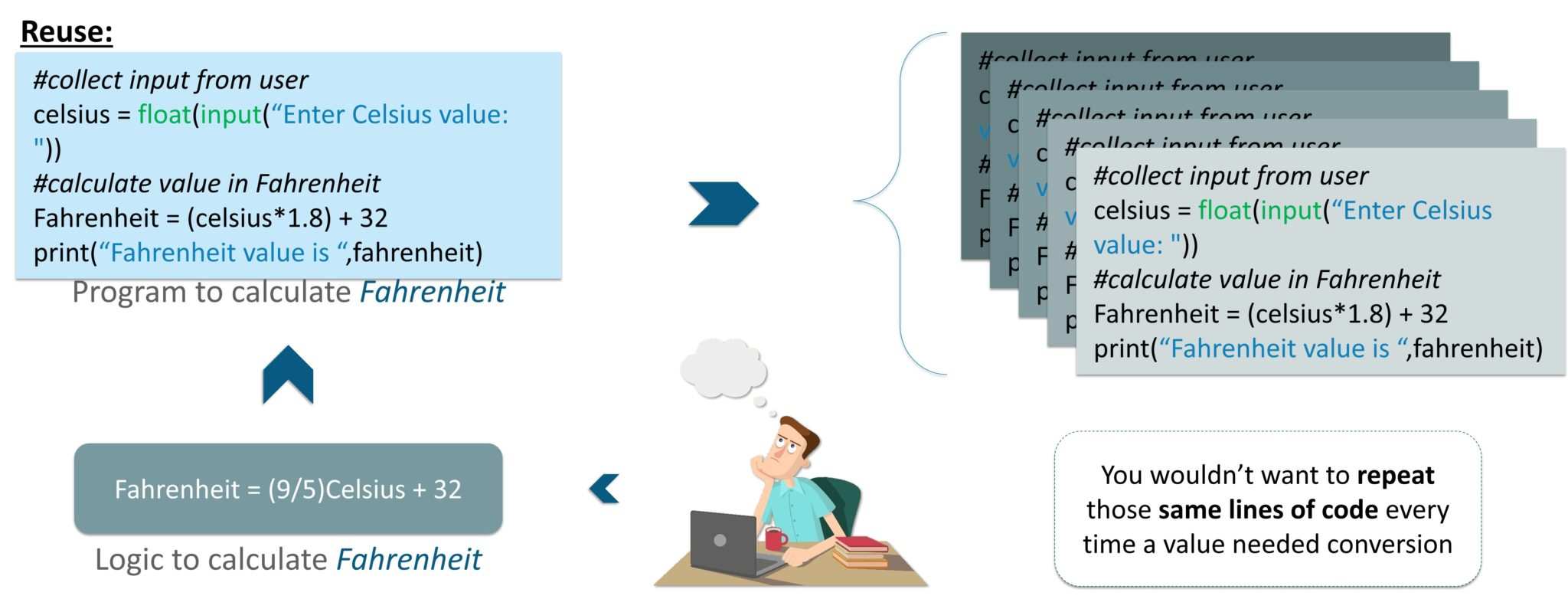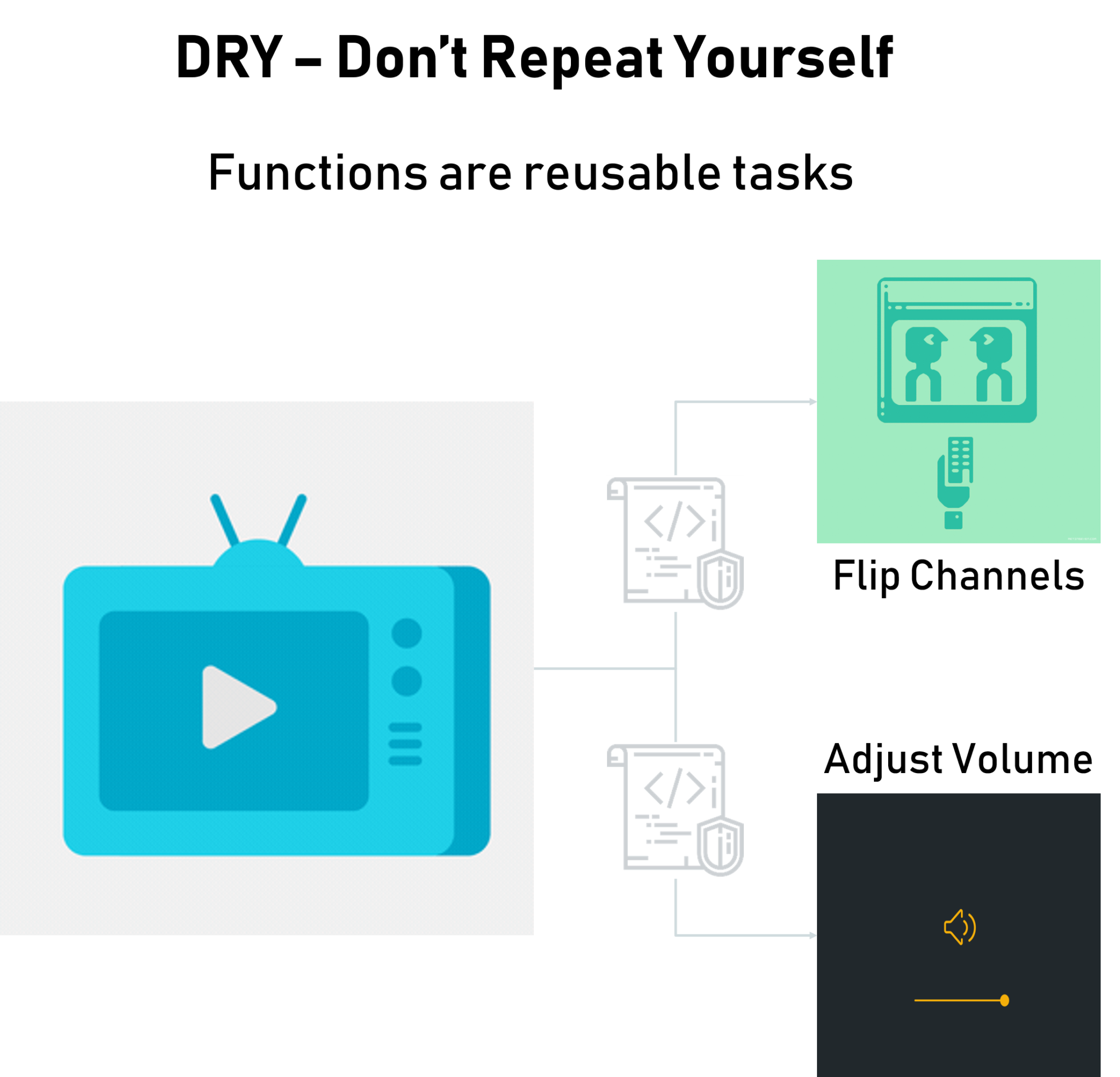{{announcement.body}}
{{announcement.title}}

# Python Functions Tutorial: Working With Functions in Python, Part 1

DZone 's Guide to

# Python Functions Tutorial: Working With Functions in Python, Part 1

### In this Python functions post, the goal is to get you the expertise required to get started and work with functions using Python. Let's get started!

· Big Data Zone ·
Free Resource

Comment (0)

Save
{{ articles.views | formatCount}} Views

In today's fast-paced IT world, it is always an advantage to have an edge over the others in terms of in-depth knowledge of a certain technology. Python is a widely used language and provides 'n' number of opportunities to enthusiastic learners.

In this Python functions post, the goal is to get you the expertise required to get started and work with functions using Python. I will be covering the following topics in this Python functions article:

• Why We Need Python Functions

• What Python Functions Are

• Types of Python Functions

• Built-in Functions in Python

• Python Recursive Functions

• Python Lambda Functions

• User-defined Functions in Python

## Why We Need Python Functions

Functions manage the inputs and outputs in computer programs. Programming languages are designed to work on data and functions are an effective way to manage and transform this data.

The modifications are generally done to drive outcomes like performing tasks and finding results. And, the set of operations or instructions required to do so comes from logically functional blocks of code that can be reused independently from the main program.

In fact, the main code is also a function, just a very important one. Every other function is logically aligned and maintained to functionally execute from your main code. But, if the function has not been defined, you'll just have to define one yourself before using it. This is because the definition lists the steps of its operation.Would you rather write a single piece of code 10 times or just once and use it 10 times?

So, functions are nothing but tasks that a user wants to perform. But, defining it once with a name will let you reuse that functionality without making your main programs look too scary. This drastically reduces lines of code and even makes debugging easier.

We'll be getting into that shortly, but the first reason behind why we use functions is because of their reusability. The fact that even complex operations could be put together as singular tasks that would run with just a call by its name is what has made code today so much clearer as well.

Every programming language lets you create and use these functions to perform various tasks with just a call. And, you could call it any number of times without having to worry about logically structuring its code into your main code every single time.Say, you have a television that stores many channels on it, receives their digital radio broadcasts, converts them into what we watch, while also giving us additional options for a variety of other features as well.

But that doesn't mean there is someone logically scripting the lines of codes for what you watch every time you turn on your tv or flip a channel. Rather, functions for each task have been logically defined once and keep getting reused time and again according to the features you try to use.

All of this happens by calling different functions as many times as needed from the main function that is running. So, even if you're turning the volume up or down, its defined function is being called repeatedly.

And, having a system operating the main code to keep calling these functions as and when needed has also made designing and innovating upon it easier.

The important thing to note is that whenever this function is called, it executes its tasks depending on the instructions specified in it.

That's why machines can have different functions. A calculator is probably the most common example of this. It has provisions for addition, subtraction, multiplication, division, and other functions. All its functions have been clearly predefined into it, but it only performs those that you choose to call by pressing a respective button.

Programmers reduce coding time and debugging time, thereby reducing overall development time, by using functions.

Next up on this Python functions post, let's look at what Python functions actually are.

## What Are Python Functions?

The functions in Python are a classic example of the reusability discussed above. So, to serve a wide range of applications from GUI and mathematical computing to web development and testing, Python's interpreter already comes equipped with numerous functions that are always available for use. And, you could also bring in other libraries or modules to your program that contain pre-defined functions readily available for use.

So, once defined, a function can be used any number of times at any point in any of your code. Now, this is because Python falls in-line with the DRY principle of software engineering, which aims to replace any repetition of software patterns or codes with abstractions to avoid redundancy and ensure that they can be used freely without revealing any inner details of their implementation.

DRY stands for Don't Repeat Yourself and this concept of having reusable blocks of codes is very crucial for achieving abstraction in Python. Thus, in order to use a function, all that you'll really need is its name, its purpose, its arguments (if it takes any), and its result's type (if it returns any).

It's almost like using an automobile or a telephone, where you don't necessarily need to understand the working of its components to use them. Rather, they've been built to serve common purposes that you can just use directly to achieve your goals and devote your precious time to implementing all the innovative aspects of your application.

A function can be called as a section of a program that is written once and can be executed whenever required in the program, thus giving us code reusability.

The function is a subprogram that works on data and produces some output.

To define a Python function, you'd have to use the `def` keyword before the name of your function and add parentheses to the end, followed by a colon.

Python uses indentation to indicate blocks, instead of brackets, to make code more readable.

A function in Python may contain any number of parameters or none. So, when you need your function to operate on variables from other blocks of code or from your main program, it could take any number of parameters and produce results.

A Python function could also optionally return a value. This value could be a result produced from your function's execution or even be an expression or value that you specify after the keyword '`return`.' And, after a return statement is executed, the program flow goes back to the state next to your function call and gets executed from there.

So, to call a Python function at any place in your code, you'll only have to use its name and pass arguments in its parentheses, if any.

The rules for naming a function are the same as naming a variable. It begins with either letter from A-Z, a-z in both upper and lower cases or an underscore(`_`). The rest of its name can contain underscores(`_`), digits(0-9), and any letters in upper or lower case.

1. A reserved keyword may not be chosen as an identifier.
2. Good usage of grammar to ensure enhanced readability of code.

It is good practice to name a Python function according to what it does.

Next up in this Python functions post, let us check out the types of functions available in Python.

## Types of Python Functions

There are many types of Python functions. And each of them is very vital in its own way. The following are the different types of Python Functions:

• Python Built-in Functions.
• Python Recursion Functions.
• Python Lambda Functions.
• Python User-defined Functions.

Let us check out these functions in detail. Beginning with Built-in functions as they are very easy to understand and implement.

## Python's Built-In Functions

The Python interpreter has a number of functions that are always available for use. These functions are called built-in functions. For example, the `print()` function prints the given object to the standard output device (screen) or to the text stream file.

In Python 3.6, there are 68 built-in functions. But for the sake of simplicity, let's consider the most popular functions and we can build up our knowledge from there.

### Python abs() Function

Definition

The `abs()` function returns the absolute value of the given number. If the number is a complex number, `abs()` returns its magnitude.

Syntax

The syntax of the `abs()` is:

``abs(num)``

Parameters

The `abs()` function takes a single argument:

1. integer
2. floating number
3. complex number

Example

``````# random integer
integer = -20
print('Absolute value of -20 is:', abs(integer))

#random floating number
floating = -30.33
print('Absolute value of -30.33 is:', abs(floating))``````

Output

``Absolute value of -20 is: 20 Absolute value of -30.33 is: 30.33``

### Python all() Function

Definition

The `all()` function returns `True` when all elements in the given iterable are true. If not, it returns `False`.

Syntax

The syntax of the `all()` is:

``all(iterable)``

Parameters

The `all()` function takes a single parameter:

Example

``````# all values true
l = [1, 3, 4, 5]
print(all(l))

# all values false
l = [0, False]
print(all(l))

# one false value
l = [1, 3, 4, 0]
print(all(l))

# one true value
l = [0, False, 5]
print(all(l))

# empty iterable
l = []
print(all(l))``````

Output

``True False False False True``

## Python ascii() Function

Definition

The `ascii()` function returns a string containing a printable representation of an object. It escapes the non-ASCII characters in the string using \x, \u or \U escapes.

Syntax

The syntax of `ascii()` is:

``ascii(object)``

Parameters

The `ascii()` function takes an object (like strings, lists, etc).

Example

``````normalText = 'Python is interesting'
print(ascii(normalText))

otherText = 'Pythön is interesting'
print(ascii(otherText))

print('Pyth\xf6n is interesting')``````

Output

``'Python is interesting' 'Pyth\xf6n is interesting' Pythön is interesting``

### Python bin() Function

Definition

The `bin()` function converts and returns the binary equivalent string of a given integer. If the parameter isn't an integer, it has to implement the `__index__()` method to return an integer.

Syntax

The syntax of the `bin()` is:

``bin(num)``

Parameters

The `bin()` function takes a single parameter:

Example

``````number = 5
print('The binary equivalent of 5 is:', bin(number))``````

Output

``The binary equivalent of 5 is: 0b101``

### Python compile() Function:

Definition

The `compile()` function returns a Python code object from the source (normal string, a byte string, or an AST object).

Syntax

The syntax of `compile()` is:

``compile(source, filename, mode, flags=0, dont_inherit=False, optimize=-1)``

Parameters

• `source`- a normal string, a byte string, or an AST object.
• `filename`- file from which the code was read. If it wasn't read from a file, you can give a name yourself.
• `mode`- Either`exec`or`eval`or`single`.
• `eval`- accepts only a single expression.
• `exec`- It can take a code block that has Python statements, classes, functions, and so on.
• `single`- if it consists of a single interactive statement.
• `flags`(optional) and`dont_inherit`(optional) - controls which future statements affect the compilation of the source. Default Value: 0.
• `optimize`(optional) - optimization level of the compiler. Default value -1.

Example

``````codeInString = 'a = 5\nb=6\nsum=a+b\nprint("sum =",sum)'
codeObejct = compile(codeInString, 'sumstring', 'exec')

exec(codeObejct)``````

Output

``sum = 11``

### Python dict() Function

Definition

`dict()` creates a dictionary in Python.

Different forms of `dict()` are:

``````class dict(**kwarg)
class dict(mapping, **kwarg)
class dict(iterable, **kwarg)``````

Example

``````numbers = dict(x=5, y=0)
print('numbers = ',numbers)
print(type(numbers))

empty = dict()
print('empty = ',empty)
print(type(empty))``````

Output

``empty = dict() print('empty = ',empty) print(type(empty))``

That's all for Part 1. Come back Monday when we'll cover even more Python functions!

Topics:
big data, python functions, python tutorial, python tutorial for beginners

Comment (0)

Save
{{ articles.views | formatCount}} Views

Published at DZone with permission of Anirudh Rao , DZone MVB. See the original article here.

Opinions expressed by DZone contributors are their own.